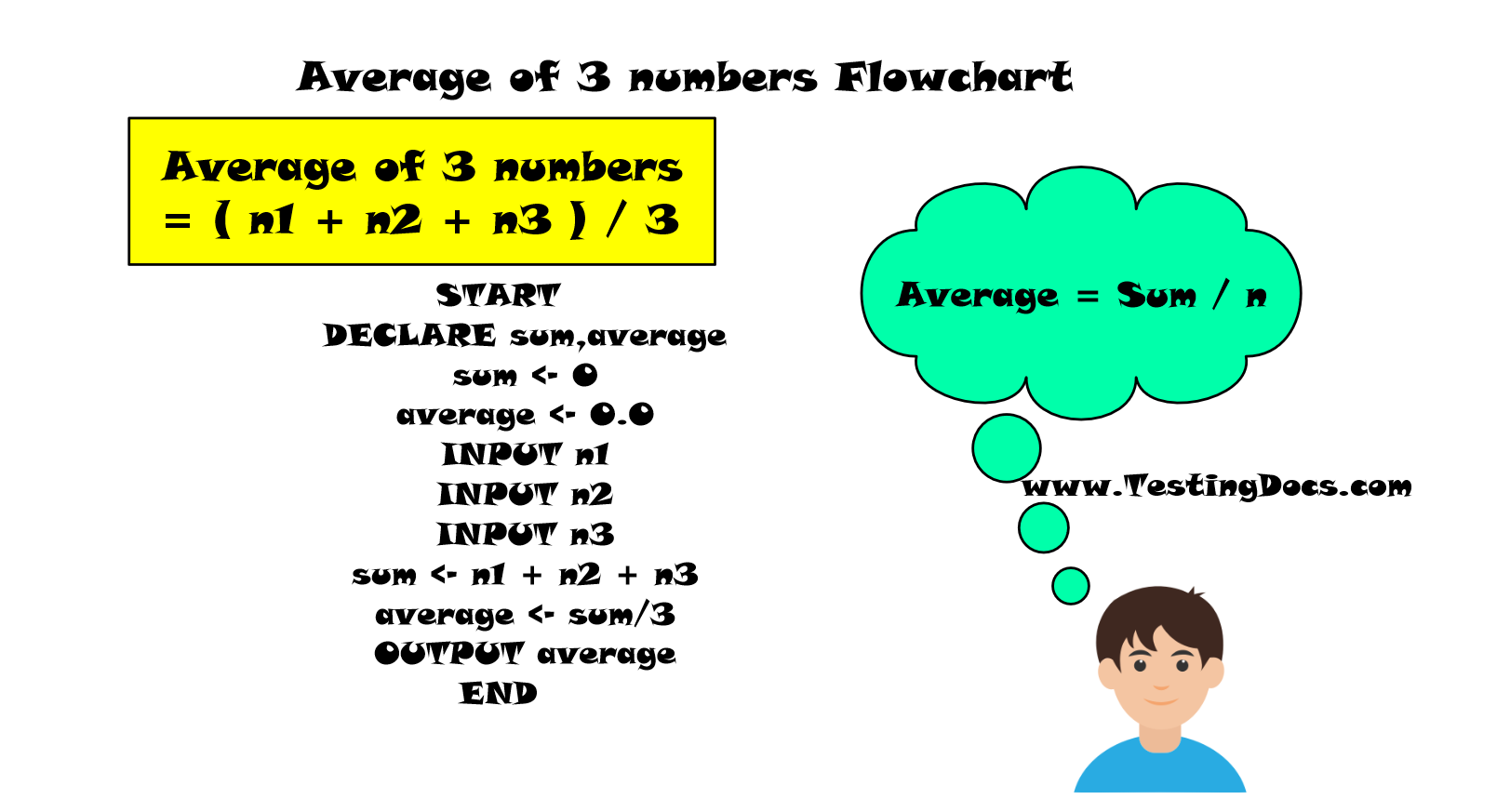# Average of three Numbers flowchart

Document Contents

### Overview

In this post, we will model a flowchart to find the average of three numbers. In general, the average of n numbers is computed as:

sum = Sum of n numbers

Here, to calculate the average of three numbers  n = 3

### Example

Let’s compute the average of the three numbers by hand. Let the three numbers be n1, n2, and n3. For example,

n1= 31,

n2 = 43

n3 = 97.

First, we need to compute the sum of the three numbers.

sum       = 31 + 43 + 97

= 171

Average = 171/ 3

= 57

### IPO chart

IPO chart for the average of three numbers flowchart

 Input Process Output Three numbers n1,n2,n3 AverageOfThree = (n1 + n2 + n3)/3 Output displayed by the flowchart AverageOfThree

###RAPTOR Flowchart

We will prompt the user to enter three numbers. In the flow chart, we will compute the average of the user entered numbers. In the end, will display the result i.e average of the three numbers.

Let the numbers are: n1, n2, and n3

We will store the average in the variable: averageOfThree

Prompt the user with the numbers.• Drag and drop the three Input symbols to the workspace.
• Compute the average :
• average = (n1 + n2 + n3 ) /3
• Drag and Drop the Assignment symbol. Perform the computation.
• Display the average.
• Drag and drop the output symbol and print the result.
• Execute the flow chart.

### Flowchart Output

Let’s execute a sample test case to test the output of the flowchart. The flowchart should be tested and the output should be validated for proper working.

 Test Case Scenario Detailed Steps Test Data Expected Result Actual Result Add three numbers. Launch Raptor. Execute the flowchart. Enter three numbers as input. 31 43 97 57We can see in the output in the MasterConsole window that the output is displayed.

### Using Arrays

To compute the average for more numbers i.e n> 3 the disadvantage of this approach is to maintain n

variables to hold the numbers. i.e n1,n2,n3 …. n

We can use an array to hold the numbers. Arrays are used to hold the variables of the same datatype.

To compute the average of more number of variables using an array:

https://www.testingdocs.com/questions/flowchart-to-calculate-total-and-average-of-array-elements/

RAPTOR tutorial page: https://www.testingdocs.com/raptor-a-flowchart-tool/

Flowchart examples: https://www.testingdocs.com/raptor-flowchart-examples/

RAPTOR official website: https://raptor.martincarlisle.com/